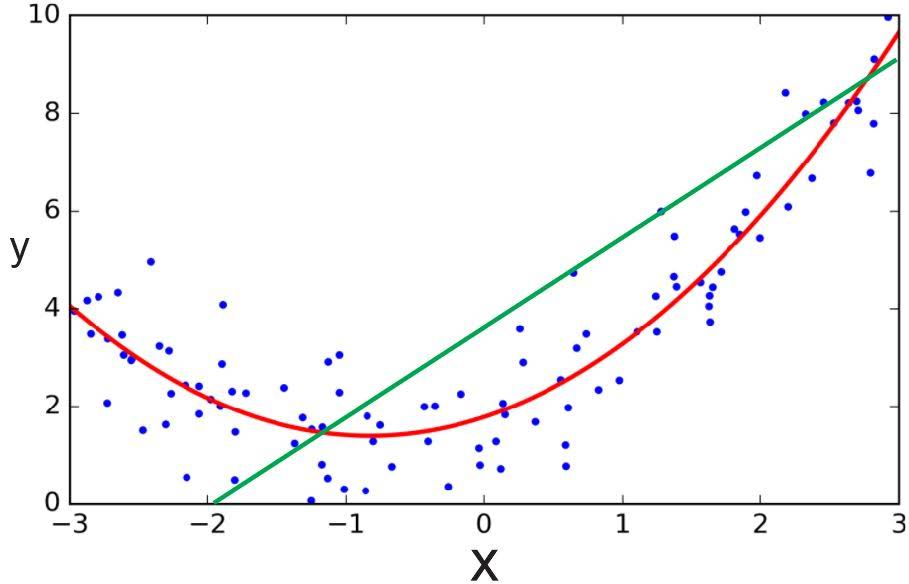# Polynomial Regression

Categories:

Updated:

Our hypothesis function need not be linear (a straight line) if that does not fit the data well.

We can change the behavior or curve of our hypothesis function by making it a quadratic, cubic or square root function (or any other form).Linear hypothesis(green line) doesn’t fit the data well. After adding quadratic features, new quadratic hypothesis(red line) fits the data better.

We are basically creating new features by combining features in polynomial forms to fit the data better.

For example, if our hypothesis function is $h_\theta(x) = \theta_0 + \theta_1 x_1$, then we can create additional features based on $x_1$, to get the quadratic function $h_\theta(x) = \theta_0 + \theta_1 x_1 + \theta_2 x_1^2$ or the cubic function $h_\theta(x) = \theta_0 + \theta_1 x_1 + \theta_2 x_1^2 + \theta_3 x_1^3$.

If you choose to use polynomial regression, then feature scaling becomes very important as the ranges of each polynomial feature differ a lot.

Categories: